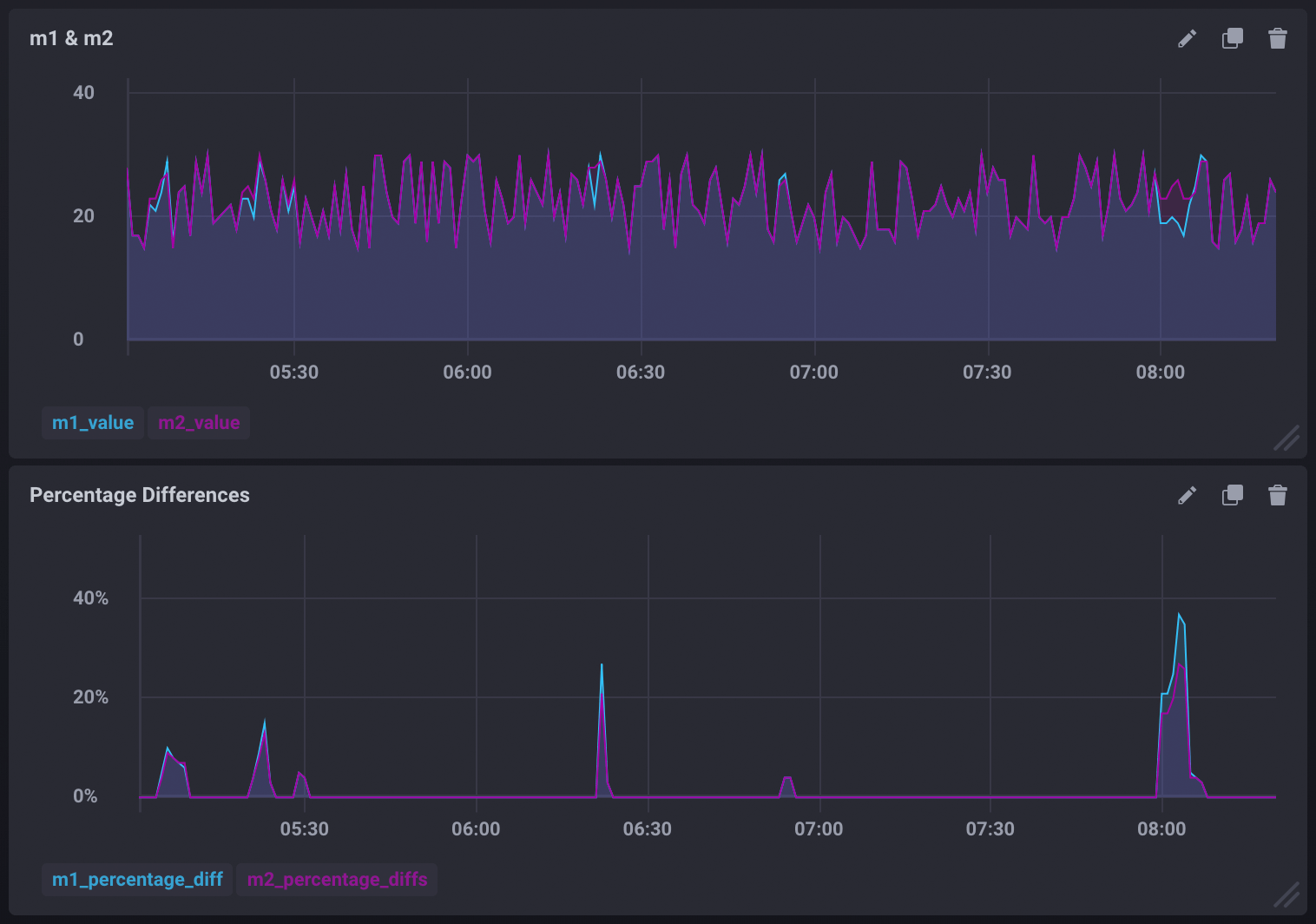# Trigger alerts by comparing two measurements

Kapacitor allows you to create alerts based on two or more measurements. In this guide, we are going to compare two measurements, `m1` and `m2`, and create an alert whenever the two measurements are different. As an added bonus, we’ll also include a query that can be used to graph the percentage difference between the two measurements.

## Comparing measurements and creating an alert

The following TICKscript streams the `m1` and `m2` measurements, joins them, compares them, and triggers an alert if the two measurements are different.

``````var window_size = 1m

// Stream m1
var m1 = stream
|from()
.measurement('m1')
|window()
.period(window_size)
.every(window_size)
.align()
|count('value')
.as('value')

// Stream m2
var m2 = stream
|from()
.measurement('m2')
|window()
.period(window_size)
.every(window_size)
.align()
|count('value')
.as('value')

// Join m1 and m2
var data = m1
|join(m2)
.as('m1', 'm2')

// Compare the joined stream and alert when m1 and m2 values are different
data
.crit(lambda: "m1.value" != "m2.value")
.message('values were not equal m1 value is {{ index .Fields "m1.value" }} m2 value is {{ index .Fields "m2.value" }}')
``````

## Graphing the percentage difference between the measurements

Use the `data` stream defined in the TICKscript above to calculate the difference between `m1` and `m2`, transform it into a float, divide that difference by the actual values of `m1` and `m2`, then multiply them by 100. This will give you the percentage difference for each. Store the difference as new fields in the `diffs` measurement:

``````data
// Calculate the difference between m1 and m2
|eval(lambda: "m1.value" - "m2.value")
.as('value_diff')
.keep()
// Calculate the % difference of m1 and m2
|eval(lambda: (float("value_diff") / float("m1.value")) * 100.0, lambda: (float("value_diff") / float("m2.value")) * 100.0)
.as('diff_percentage_m1', 'diff_percentage_m2')
// Store the calculated differences in the 'diffs' measurement
|influxDBOut()
.measurement('diffs')
.database('mydb')
.create()
``````

This can be used to create visualizations similar to:## The full TICKscript

Below is the entire, uncommented TICKscript:

``````var window_size = 1m

var m1 = stream
|from()
.measurement('m1')
|window()
.period(window_size)
.every(window_size)
.align()
|count('value')
.as('value')

var m2 = stream
|from()
.measurement('m2')
|window()
.period(window_size)
.every(window_size)
.align()
|count('value')
.as('value')

var data = m1
|join(m2)
.as('m1', 'm2')

data
.crit(lambda: "m1.value" != "m2.value")
.message('values were not equal m1 value is {{ index .Fields "m1.value" }} m2 value is {{ index .Fields "m2.value" }}')

data
|eval(lambda: "m1.value" - "m2.value")
.as('value_diff')
.keep()
|eval(lambda: (float("value_diff") / float("m1.value")) * 100.0, lambda: (float("value_diff") / float("m2.value")) * 100.0)
.as('diff_percentage_m1', 'diff_percentage_m2')
|influxDBOut()
.measurement('diffs')
.database('mydb')
.create()
``````

### Upgrade to InfluxDB Cloud or InfluxDB 2.0!

InfluxDB Cloud and InfluxDB OSS 2.0 ready for production.